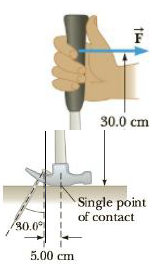Chapter 8, Problem 76AP

Chapter
Section
Textbook Problem

Figure P8.76 shows a clawhammer as it is being used to pull a nail out of a horizontal board. If a force of magnitude 150 N is exerted horizontally as shown, find (a) the force exerted by the hammer claws on the nail and (b) the force exerted by the surface at the point of contact with the hammer head. Assume that the force the hammer exerts on the nail is parallel to the nail and perpendicular to the position vector from the point of contact.Figure P8.76

(a)

To determine
The force exerted by the hammer claws on the nail.

Explanation

The force on the nail due to hammer claws is perpendicular about pivot point as shown in the figure and from the condition of static torque, τ=0 is (l/cosθ)Pl'F=0 , here the torque due to force P is taken to be positive as it is counter clockwise and the torque due to force F is negative due it is clockwise direction. By rearranging this expression for P , the force exerted by the hammer claws on the nail is calculated.

Given info: The angle force P with vertical axis is 30.0° , the horizontal distance between the pivot and the claws is 5.00cm , the force applied on handle of the hammer is 150N , and length of the hammer is 30.0cm .

The formula for the force exerted by the hammer claws on the nail,

P=l'lFcosθ

• l is the horizontal distance between the pivot and the claws.
• l' is length of the hammer

(b)

To determine
The force exerted by the surface at the point of contact with the hammer head.

Still sussing out bartleby?

Check out a sample textbook solution.

See a sample solution

The Solution to Your Study Problems

Bartleby provides explanations to thousands of textbook problems written by our experts, many with advanced degrees!

Get Started